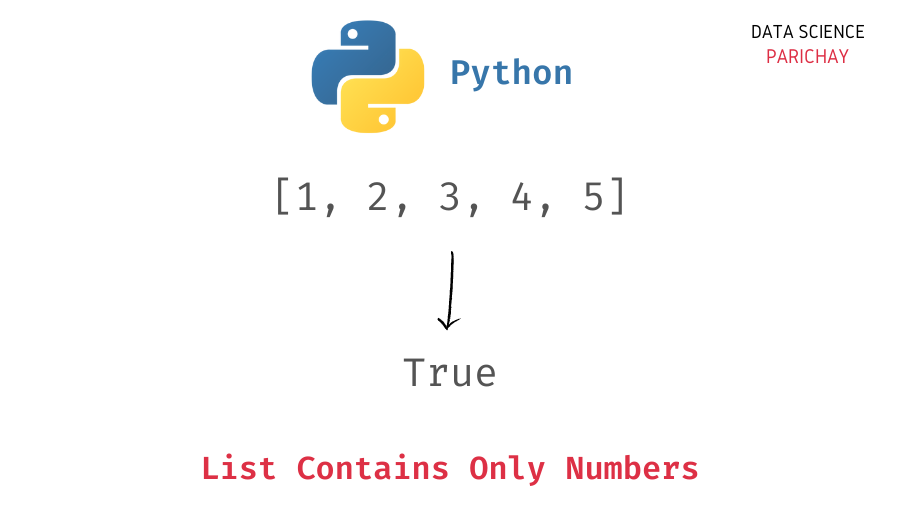# Check If a List Contains Only Numbers in Python

In this tutorial, we will look at how to check if a list contains only numbers in Python with the help of some examples.

You can use a combination of the Python built-in `isinstance()` and `all()` function to check if a list contains only numbers. For instance, you can use the following steps to check if all elements in a list are integers in Python –

1. In a list comprehension, for each element in the list, check if it’s an integer using the `isinstance()` function.
2. Apply the Python built-in `all()` function to return `True` only if all the items in the above list comprehension correspond to `True`.

The following is the code to check if all elements in a list are integers or not.

```# check if all elements in ls are integers
all([isinstance(item, int) for item in ls])```

Let’s take a look at an example.

```# list of numbers
ls = [1, 2, 3, 4]
# check if list contains only numbers
print(all([isinstance(item, int) for item in ls]))```

Output:

`True`

We get `True` as the output as all elements in the list `ls` are integers.

Let’s take a look at another example.

```# list of numbers and a string
ls = [1, 2, 3, 4, 'cat']
# check if list contains only numbers
print(all([isinstance(item, int) for item in ls]))```

Output:

📚 Data Science Programs By Skill Level

Introductory

Intermediate ⭐⭐⭐

🔎 Find Data Science Programs 👨‍💻 111,889 already enrolled

Disclaimer: Data Science Parichay is reader supported. When you purchase a course through a link on this site, we may earn a small commission at no additional cost to you. Earned commissions help support this website and its team of writers.

`False`

Here we get `False` as the output because not all elements in the list `ls` are integers. One element, “cat” in the list is a string.

If you, however, have a list of strings and want to check whether all the elements in the list are digits or not, you can use the following code.

```# list of numeric strings
ls = ["1", "2", "3", "4"]
# check if list of string contains only numberic elements
print(all([item.isdigit() for item in ls]))```

Output:

`True`

Here we check whether each element in the list of strings, `ls` is a numerical value or not using the string `isdigit()` function. We get `True` as the output as all the strings in the list `ls` are numeric characters.

You might also be interested in –

•